## The capacitor on DC voltage - an energy store

Test:

Here is the circuit diagram for a better understanding:Energy Stored on a Capacitor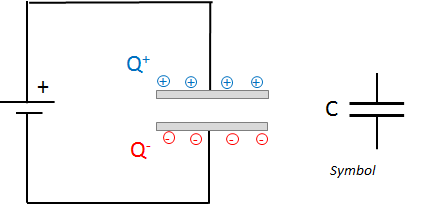Capacitor - Function and Symbol

At DC voltage, the capacitor acts as a charge accumulator. Essentially, the capacitor consists of two plates, which are electrically separated from each other - either by air or by an insulator (dielectric).

A characteristic value of the capacitor is the capacitance C. It describes the capacity of the capacitor to store a certain amount of charge Q on its plates at a certain voltage.

Q = C * V    or    C = Q / V

Colloquially expressed: "The larger the capacitor and the higher the voltage, the more charges find room on the capacitor plates".

Unit: [C] = Farad F = 1 C / V ("One Farad equals one Colomb per Volt")

Usual sizes:

How to determine the capacity of a capacitor?

Plate distance: The smaller the plate distance, the more the charges on the opposite plate attract each other and the "more" charges therefore find "space" on the plates.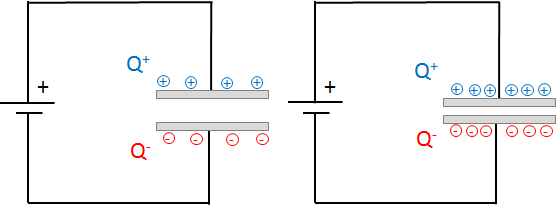Capacitor - Plate distance and capacity

Plate area A: The larger the plate areas, the more loads can be stored on the plate.
Dielectric: Electric dipoles of the dielectric align themselves with the charges of the capacitor and "neutralize" them. Thus, more charges find place on the plates of the capacitor.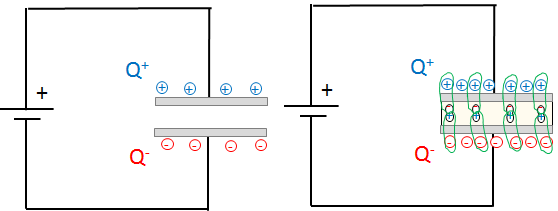Capacitor - Dielectric and Capacity

### Charge- and Discharge Curve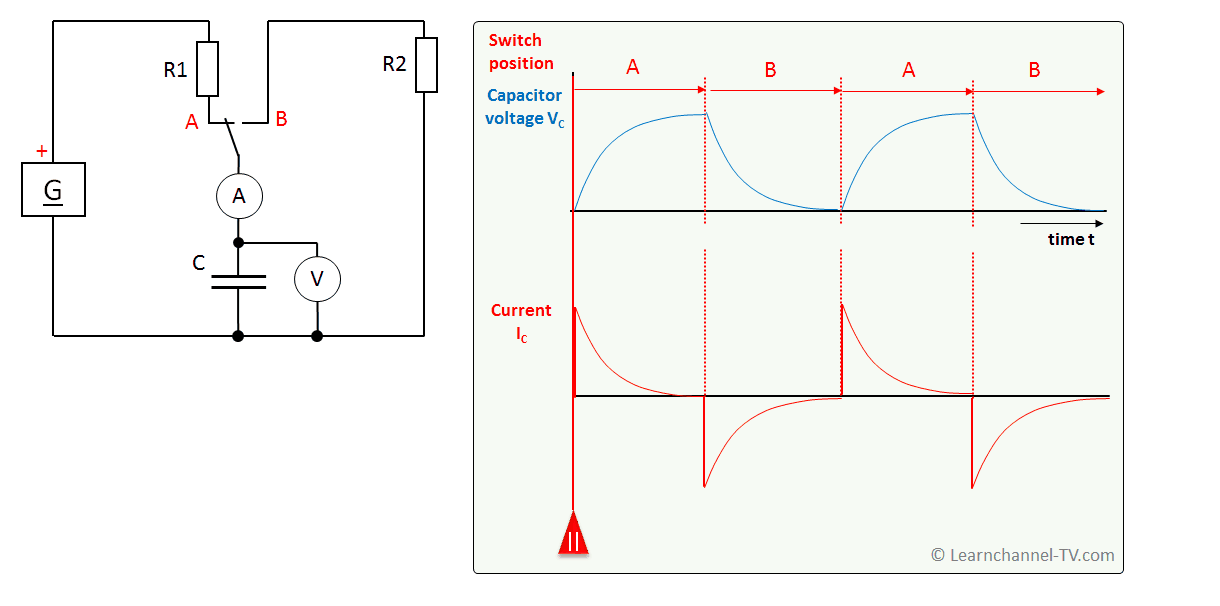Capacitor - Charge- and Discharge curve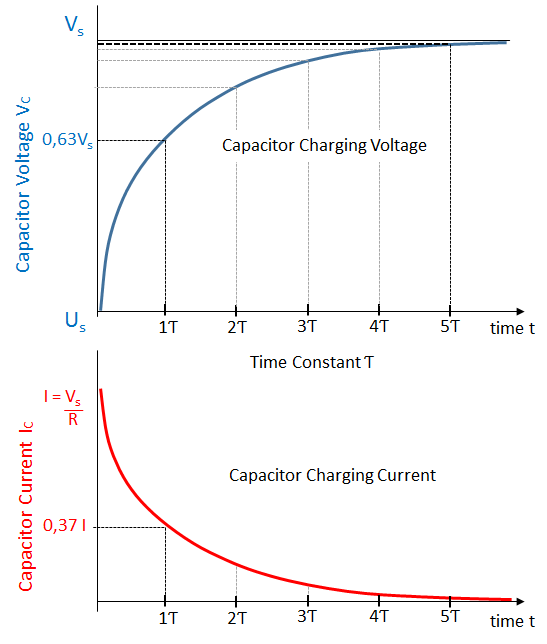Capacitor Charging Graph

Determine the time to charge or discharge a capacitor

As can be seen, the charge and discharge curves of a capacitor have a curvilinear shape, which can be described according to an e-function. Therewith, the capacitor would actually never be fully charged, a thing that of course does not make sense in practice.

In general, a capacitor charges and discharges more slowly the larger its capacity and the larger the resistor R in series. This is the basis for the so-called Time constant Ƭ (pronounced "Tau"):

Ƭ = R * C in seconds s

In practice, you would apply the following rule of thumb: The capacitor is fully charged or discharged after 5 Ƭ. The capacitor voltage has thus reached 0.99 of the supply voltage.

The level of the applied voltage has no influence on the charging time!

Always charge and discharge capacitors - especially larger ones - via a series resistor!

### Capacitor Circuits

Capacitor in SeriesCapacitor in Series

As can be seen in the picture, the plate distances add up when capacitors are connected in series. This means that the total capacitance must be reduced:

1       =   1   +  1  +    1   +  …
CEq      C1      C2      C3

Capacitor in Parallel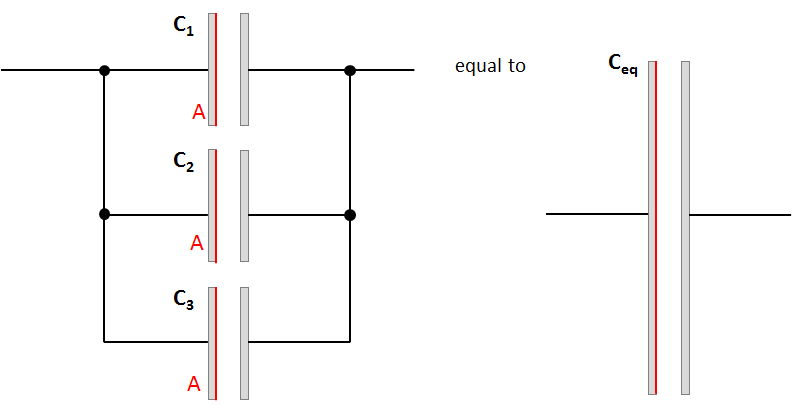Capacitor in Parallel

As can be seen in the picture, when capacitors are connected in parallel, the plate surfaces add up. Therefor the total capacitance increases:

CEq  =  C1 + C2 + C3 + …